# 1st PUC Physics Question Bank Chapter 11 Thermal Properties of Matter

## Karnataka 1st PUC Physics Question Bank Chapter 11 Thermal Properties of Matter

### 1st PUC Physics Thermal Properties of Matter Textbook Questions and Answers

Question 1.
The triple points of neon and carbon dioxide are 24.57 K and 216.55 K respectively. Express these temperatures on the Celcius and Fahrenheit scales.
Tc = T – 273.15
∴ Tc for neon = 24.57 – 273.15
= – 248.58°C
and Tc for carbon dioxide
= 216.55 – 273.15
= – 56.60°C
tF = $$\frac{9}{5}$$ tc + 32
∴ tF for neon = $$\frac{9}{5}$$ × (-248.58) + 32
= – 415.44°C
tF for carbon dioxide
= $$\frac{9}{5}$$ × (-56.60) + 32
= – 69.88°F

Question 2.
Two absolute scales A and B have triple points of water defined to be 200A and 350B. What is the relation between TA and TB?
Triple point of water
= 273.16 K = 200A
= 350B
∴ Size of 1K on scale A = $$\frac{273.16}{200}$$
and size of 1K on scale B = $$\frac{273.16}{350}$$
Value of temperature TA on scale A = $$\frac{273.16}{200}$$ TA
Value of temperature TB on scale B = $$\frac{273.16}{350}$$ TB
Since the two temperatures are equal,
$$\left(\frac{273.16}{200}\right) \mathrm{T}_{\mathrm{A}}=\left(\frac{273.16}{350}\right) \mathrm{T}_{\mathrm{B}}$$
⇒ TA = $$\frac{4}{7}$$ TBQuestion 3.
The electrical resistance in ohms of a certain thermometer varies with temperature according to the approximate law: R = R0 [1 + α (T – T0)] The resistance is 101.6Ω at the triple point of water 273.16K, and 165.5 Ω at the normal melting point of lead (600.5K). What Is the temperature when the resistance is 123.4 Ω?
At T0 = 273.16K, R0= 101.6 Ω
At T1 = 600.5K, R1 = 165.5 Ω
Let T2 be the temperature at which the resistance is R2 = 123.4 Ω
R = R0 [1 + α (T – T0)]
⇒ T – T0 = $$\frac{\mathrm{R}-\mathrm{R}_{0}}{\alpha \mathrm{R}_{0}}$$
∴ T1 – T0 = $$\frac{\mathrm{R}_{1}-\mathrm{R}_{0}}{\alpha \mathrm{R}_{0}}$$→ (1)
T2 – T0 = $$\frac{\mathrm{R}_{2}-\mathrm{R}_{0}}{\alpha \mathrm{R}_{0}}$$→ (2)
Dividing (2) by (1) gives,
$$\frac{\mathrm{T}_{2}-\mathrm{T}_{0}}{\mathrm{T}_{1}-\mathrm{T}_{0}}=\frac{\mathrm{R}_{2}-\mathrm{R}_{0}}{\mathrm{R}_{1}-\mathrm{R}_{0}}$$
$$\mathrm{T}_{2}=\mathrm{T}_{0}+\left(\mathrm{T}_{1}-\mathrm{T}_{0}\right)\left(\frac{\mathrm{R}_{2}-\mathrm{R}_{0}}{\mathrm{R}_{1}-\mathrm{R}_{0}}\right)$$
= 293.16 + (600.5-273.16) $$\left(\frac{123.4-101.6}{165.5-101.6}\right)$$
⇒ T2 = 384.83 K

Question 4.
1. The triple point of water is a standard fixed point in modern thermometry. Why? What is wrong in taking the melting point of ice and the
boiling point of water as standard fixed points (as was originally done in the Celcius scale)?

2. There were two fixed points in the original Celcius scale as mentioned above which were assigned the number 0°C and 100°C respectively. On the absolute scale, one of the fixed points is the triple point of water, which on the Kelvin absolute scale is assigned the number 273.16K. What is the other fixed point on this (Kelvin) scale?

3. The absolute temperature (Kelvin scale) T is related to the temperature te on the Celcius scale by tc = T – 273.15 Why do we have 273.15 in this relation and not 273.16?

4. What is the temperature of the triple point of water on an absolute scale whose unit interval size is equal to that of the Fahrenheit scale?
1. The triple point is used as a standard because of the unique conditions of temperature and pressure at this point. The melting point of ice and boiling point of water depend on pressure. So they cannot be used as reference points.

2. The other fixed point is absolute zero, the theoretically lowest possible temperature.

3. We have 273.15 in the relation and not 273.16 because the triple point of water is 0.01 °C and not 0°C.
In other words, 0.01 °C → 273.16 K
⇒ 0°C → 273.15 K
∴ tc = T – 273.15

4. The unit interval size in the Fahrenheit scale = $$\frac{212-32}{100}$$ =1.8
∴ The triple point of water = 273.16 × 1.8
= 491.69

Question 5.
Two ideal gas thermometers A and B use oxygen and hydrogen respectively. The following observations are made: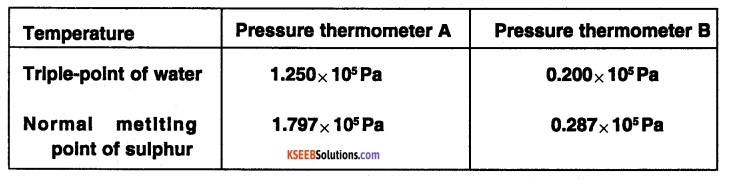1. What is the absolute temperature of normal melting point of sulphur as read by thermometers A and B?
2. What do you think Is the reason behind the slight difference in the answers of thermometers A and B? (The thermometers are not faulty) What further procedure is needed In the experiment to reduce the discrepancy between the two readings?
1. Let P be the pressure in the pressure thermometer and T be the corresponding absolute temperature.
Let P0 and T0 be the values for the triple point of water.
Then $$\frac{T}{T_{0}}=\frac{P}{P_{0}}$$
$$\therefore \frac{T_{A}}{273.16}=\frac{1.797 \times 10^{5}}{1.250 \times 10^{5}}$$
⇒ TA = 392.69 K
$$\frac{T_{B}}{273.16}=\frac{0.287 \times 10^{5}}{0.200 \times 10^{5}}$$
⇒ TB= 391.98 K

2. The discrepancy is due to the non¬ideal behaviour of the gases. To reduce this discrepancy, measurements at lower pressures should be taken.

Question 6.
A steel tape 1m long Is correctly calibrated for a temperature of 27.0°C. The length of a steel rod measured by this tape Is found to be 63.0 cm on a hot day when the temperature is 45.0°C. What is the actual length of the steel rod on that day? What is the length of the same steel rod on a day when the temperature is 27.0° C? Coefficient of linear expansion of steel = 1.20 × 10-5K-1.
l0 = 63 cm
∆T = 45°C – 27°C .
= 18°C = 18 K
α = 1.2 × 10-5K-1
l = l0 (1 + α ∆T)
= 63(1 + 1.2 × 10-5 × 18)
l = 63.0136 cm.
∴ Actual length of the steel rod at 45°C =63.0136 cm
At 27°C, the steel tape is correctly calibrated.
∴ the length of the steel rod = 63.0 cmQuestion 7.
A large steel wheel Is to be fitted on to a shaft of the same material. At 27°C, the outer diameter of the shaft is 8.70 cm and the diameter of the central hole In the wheel is 8.69 cm. The shaft Is cooled using ‘dry ice’. At what temperature of the shaft does the wheel slip on the shaft? Assume the coefficient of linear expansion of the steel to be constant over the required temperature range: αsteel = 1.2 × 10-5K-1.
Given αsteel = 1.2 × 10-5K-1
l1 = 8.70 cm
l2 = 8.69 cm (because the shaft is cooled to help it slip on the wheel)
T1 = 27°C = 300 K
T2 =?
l2 = l1 [1 + αsteel(T2 – T1)]
⇒ 8.69 = 8.70 [1 + 1.2 × 10-5(T2 – 300)]
⇒ 1 + 1.2 × 10-5(T2 – 300) = $$\frac{8.69}{8.70}-1$$
⇒ T2 = 300 + $$\frac{-0.01}{8.70} \times \frac{1}{1.2 \times 10^{-5}}$$
= 204.21 K
= – 68.94°C
= – 69°C
∴ The required temperature = – 69°C

Question 8.
A hole drilled in a copper sheet. The diameter of the hole is 4.24cm at 27.0°C. What is the change in the diameter of the hole when the sheet is heated to 227° C? Coefficient of linear expansion of copper = 1.70 × 10-5K-1.
Here, L = diameter of hole = 4.24 cm
ΔT = 227 – 27 = 220 °C and α = 1.70 x 10-5 C-1
Change in diameter,
ΔL = aLΔT = 1.70 x 10-5 x 4.24 x 200 = 1.44 x 10-2 cm.

Question 9.
A brass wire 1.8 m long at 27°C Is held taut with little tension between two rigid supports. If the wire Is cooled to a temperature of -39°C, what Is the tension developed In the wire, if its diameter is 2.0 mm?
Coefficient of linear expansion of brass = 2.0 × 10-5 K-1, Young’s modulus Of brass = 0.91 × 1011 Pa.
l = 1.8 m,
T1 =27°C = 300 K,
T2 = – 39°C =234 K,
d = 2mm = 2 × 10-3
αbrass = 2.0 × 10-5 K-1,
Ybrass = 0.91 × 1011 Pa
$$\frac{\Delta \ell}{\ell}=\alpha \Delta \mathrm{t}$$
$$Y=\frac{\Delta F / A}{\Delta \ell / \ell}=Y\left(\frac{\Delta \ell}{\ell}\right) A$$
= Y(α ∆T) $$\pi\left(\frac{\mathrm{d}}{2}\right)^{2}$$
= 0.91 × 1011 × 2.0 × 10-5 α (234 – 300) × π × $$\left(\frac{2 \times 10^{-3}}{2}\right)^{2}$$
= – 377.37 N
Tension developed ∆F = 380N (positive value, rounded to 2 significant figures)

Question 10.
A brass rod of length 50cm and diameter 3.0 mm is Joined to a steel rod of the same length and diameter. What is the change in length of the combined rod at 250°C, if the original lengths are at 40.0°C? Is there a ‘thermal stress’ developed at the junction? The ends of the rod are free to expand (Coefficient of linear expansion of brass = 2.0 × 10-5K-1, steel = 1.2 × 10-5K-1).
Given:  lbrass = 50cm, lsteel = 50cm
T1 = 40°C = 313K
T2 = 250°C = 523 K
αbrass = 2.0 × 10-5K-1
αsteel = 1.2 × 10-5K-1
∆lbrass = lbrass × αbrass × ∆T
= 50 × 2.0 × 10-5 × (523 – 313)
∆lbrass = 0.21 cm
∆lsteel = lsteel × αsteel × ∆T
= 50 × 1.2 × 10-5 × (523 – 313)
= 0.126 cm
∆lsteel = 0.13cm
(round off to two significant figures) Since the rods are free to expand, no thermal stress is developed at the junction.

Question 11.
The coefficient of volume expansion of glycerin is 49 × 10-5K-1. What is the fractional change in its density for a 30°C rise In temperature?
Given, rglycerin = 49 × 10-5K-1
∆T = 30°C = 30 K
Let the volume be V1 at temperature and V2 at temperature T2
Using V2 = V1(1 + r ∆T), we get
$$\frac{V_{1}}{V_{2}}=\frac{1}{1+r \Delta T}$$
$$=\frac{1}{1+49 \times 10^{-5} \times 30}=0.9855$$
Fractional change in density= 0.9855 – 1
= – 0.0145
= – 1.5 × 10-2
(The negative sign denotes a decrease in density).Question 12.
A 10 kW drilling machine is used to drill a bore In a small aluminium block of mass 8.0 kg. How much is the rise in temperature of the blocks in 2.5 minutes, assuming 50% of power is used up in heating the machine itself or lost to the surroundings? Specific heat of aluminium = 0.91 J g-1 K-1
Given,
P = 10kW,
t = 2.5 minutes = 150s,
m = 8 kg,
saluminium = 0.91 Jg-1 K-1 = 910 Jkg-1 K-1
Energy transferred to the aluminium
= 50% of p × t
This energy heats up the aluminium.
So, $$\frac{50}{100}$$ × pt = m saluminum ∆T
⇒ $$\frac{1}{2}$$ × 10 × 103 × 150 = 8 × 910 × ∆T
⇒ ∆T = 103 K
or ∆T = 103°C

Question 13.
A copper block of mass 2.5kg is heated in a furnace to a temperature of 500°C and then placed on a large ice block. What is the maximum amount of ice that can melt? (Specific heat of copper = 0.39 Jg-1 K-1 heat of fusion of water = 335 Jg-1)
Given mcopper = 2.5 kg,
T1= 500°C,
T2 = 0°C,
Scopper = 0-39 Jg-1 K-1
Lice = 335 Jg-1
Let the mass of ice that melts be mice
Heat dissipated by the copper block = Heat absorbed by the ice.
⇒ mcopper Scopper ∆T = mice Lice
⇒ 2500 × 0.39 × (500 – 0) = mice × 335
⇒ mice = 1455 g
⇒ mice = 1.5 kg
(rounding off to 2 significant figures)

Question 14.
In an experiment on the specific heat of a metal, a 0.20 kg block of the metal at 150°C Is dropped in a copper calorimeter (of water equivalent 0.025 kg) containing 150 cm3 of water at 27°C. The final temperature is 40°C. Compute the specific heat of the metal. If heat losses to the surroundings are not negligible, is your answer greater or smaller than the actual value of the specific heat of the metal?
Given, mass of metal block,
m1 = 0.20 kg
Initial temperature of the metal block,
T1 = 150°C
Initial temperature of the calorimeter and water,
T2 = 27°C
Final temperature of the mixture,
T3 = 40°C
Volume of water in the calorimeter,
V =150 cm3
= 150 × 10-6 m3
Mass of the water = Density × Volume
= 1000 × 150 × 10-6
= 150 × 10-3 kg
Let s1 be the specific heat of the metal.
s2 is the specific heat of water.
Water equivalent of the calorimeter,
W = 0.025 kg
Heat lost by the metal block = Heat gained by the calorimeter and water.
⇒ m1 s1 ∆T1 = (m2 + W) s2 ∆T2
⇒ 0.2 × s1 × (150 – 40)
= (150 × 10-3 + 0.025) × 4200 × (40 – 27)
⇒ s1 = 434.3 J kg-1K-1
= 430 J kg-1K-1
or 0.43 Jg-1K-1
If the heat losses to the surroundings are not negligible, the left-hand side of the expression would have had an extra term added to it to account for this loss. Upon solving for s2 we would get a smaller value than the actual value.

Question 15.
Given below are observations on molar specific heats at room temperature of some common gases.
Gas                         Molar specific heat (cv) (cal mol’1 K’1)
Hydrogen               4.87
Nitrogen                 4.97
Oxygen                   5.02
Nitric oxide             4.99
Carbon monoxide   5.01
Chlorine                  6.17
The measured molar specific heats of these gases are markedly different from those for monoatomic gases. Typically, the molar specific heat of a monoatomic gas is 2.92 cal/mol K. Explain this difference. What can you Infer from the somewhat larger (than the rest) value for chlorine?
The measured molar specific heats of the gases mentioned in the table are markedly different because all these gases are diatomic gases. A monoatomic gas has only translational motion. In a diatomic gas, apart from translational motion, vibrational as well as rotational motion is also possible. Therefore, to increase the temperature of this gas through 1°C, an extra amount of heat is required to increase the average energy of different modes of motion. Under ordinary temperature conditions (say room temperature) diatomic gases usually have rotational motion apart from their translational motion. But for chlorine, the vibrational motion also occurs. This is the reason that chlorine has a somewhat larger value of molar-specific heat.Question 16.
Answer the following questions based on the P-T diagram of carbon dioxide:
1. At what temperature and pressure can the solid, liquid and vapour phases of Co2, exist in equilibrium?
2. what is the effect of a decrease of pressure on the fusion and boiling point of Co2?
3. What are the critical temperature and pressure for Co2? What is their significance?
4. Is CO2 solid, liquid or gas at

• – 70°C under 1 atm,
• – 60°C under 10 atm,
• 15°C under 56 atm?1. At -56.6°C and 5.11 atm, which is the triple point of Co2, all 3 .phases can coexist in equilibrium.
2. From the fusion and vaporisation curves, we see that reducing the pressure lowers the fusion and boiling point.
3. The critical temperature and pressure are 31.1°C and 73.0 atm respectively. Above this temperature, Co2 will not liquefy even if compressed to high pressures.
4.

• Gas
• Solid
• Liquid

Question 17.
Answer the following questions based on the P-T phase diagram of Co2:

1. CO2 at 1 atm pressure and temperature – 60°C is compressed isothermally. Does it go through a liquid phase?
2. What happens when Co2 at 4 atm pressure is cooled from room temperature at constant pressure?
3. Describe qualitatively the changes In a given mass of solid Co2 at 10 atm pressure and temperature -65°C as it is heated up to room temperature at constant pressure.
4. CO2 is heated to a temperature of 700C and compressed isothermally. What changes in its properties do you expect to observe?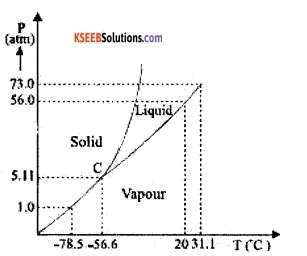(a) Since – 60° lies to left of – 56.6 °C on the curve, the vapour will condense directly into the solid.

(b) Since 4 atm pressure is less than 5-11 atm, the vapour will condense directly into the solid without entering into the liquid region.

(c) When the solid CO2 at – 65 °C is heated at 10 atm pressure, it is first converted into a liquid. A further increase in its temperature brings it into the vapour phase. If a horizontal line at P = 10 atm is drawn parallel to the T­axis, then the points of intersection of this line with the fusion and vaporization curve give the fusion and boiling points at 10 atm.

(d) Above 31.1°C, the gas can not be liquefied. Therefore, on being compressed isothermally at 70°C, there will be no transition to the liquid region. However, the gas will depart more and more from its perfect gas behaviour with the increase in pressure.

Question 18.
A child running a temperature of 101°F is given an antipyrin (i.e., a medicine that lowers fever) which causes an increase in the rate of evaporation of sweat from his body. If the fever is brought down to 98°F in 20 min, what is the average rate of extra evaporation caused by the drug? Assume the evaporation mechanism to be the only way by which heat is lost. The mass of the child is 30 kg. The specific heat of the human body is approximately the same as that of water, and the latent heat of evaporation of water at that temperature is about 580 cal g-1.
Mass of the child, m = 30 kg
Change in temperature ∆T ,
= 101°F – 98°F
= 3°F
= 3 × 5/9 = 5/3°c
Specific heat of water,
s = 4.2 × 103 J kg-1°C-1
Latent heat of vaporisation,
L = 580 cal g-1
= 580 × 4.2 × 103 J kg-1
Time taken for the sweat to evaporate,
t = 20 min.
Let m1 be the mass of sweat that evaporates.
Now, heat lost by the child during evaporation
= Heat needed for the sweat to evaporate.
⇒ ms ∆T = m1L
⇒ 30 × 4.2 × 103 × 5/3
= m1 × 580 × 4.2 × 103
⇒ m1 = 0.0862 kg
m1 = 86.2 g
Rate of extra evaporation caused by the drug = Rate of evaporation of sweat
$$=\frac{m_{1}}{t}=\frac{86.2 g}{20 m i n}$$
= 4.3 g min-1

Question 19.
A ‘thermacole’ icebox is a cheap and efficient method for storing small quantities of cooked food In summer in particular. A cubical icebox of side 30 cm has a thickness of 5.0 cm. If 4.0 kg pt Ice is put in the box, estimate the amount of Ice remaining after 6h. The outside temperature is 45°C, and the coefficient of thermal conductivity of thermacole is 0.01 J s-1 m-1K-1. [Heat of fusion of water = 835 × 103 J kg-1]
Total surface area of the cube,
A = 6 × (30 cm)2 = 0.54 m2
Mass of ice in the box M = 4 kg
Thickness of the box, L = 5 cm = 0.05 m
Thermal conductivity of thermacole,
k = 0.01 Js-1m-1K-1.
Outside temperature T1 = 45°C
The temperature of ice T2 = 0°C
Let the mass of ice that melts be m kg.
Heat of fusion of water,
Lf ice = 335 × 103 J kg-1
Time t = 6h = 6 × 3600 s = 21600 s
Heat gained by ice during fusion = Heat conducted through the walls of the box
⇒ m Lf, ice = $$\frac{\mathrm{KA}\left(\mathrm{T}_{1}-\mathrm{T}_{2}\right) \mathrm{t}}{\mathrm{L}}$$
⇒ m × 335 × 103
$$=\frac{0.01 \times 0.54 \times(45-0)}{0.05} \times 21600$$
⇒ m = 0.31 kg
∴ Mass of ice left in the box = M – m
= 4 – 0.31
= 3.69
= 3.7 kg.Question 20.
A brass boiler has a base area of 0.15 m2 and thickness of 1.0 cm. It bolls water at the rate of 6.0 kg/min when placed on a gas stove. Estimate the temperature of the part of the flame in contact with the boiler. Thermal conductivity of brass = 109 J s-1m-1K-1; Heat of vaporisation of water = 2256 × 103 J kg-1.
Let the temperature of the flame be T1
T2 = boiling point of water = 100°C
Thermal conductivity of brass,
k = 109 J s-1m-1K-1
Base area of the boiler, A = 0.15 m2
Thickness of the boiler, L = 1 cm = 0.01 m
⇒ Heat of vaporisation of water
Lsteam = 2256 × 103 J kg-1
Rate of boiling water
= 6 kg min-1 = $$\frac{6}{60}$$ kg s-1
= 0.1 kg s-1
∴ Power consumed = 0.1 × Lsteam
= 0.1 × 2256 × 103W
= 2256 × 102W
∴ Powe transmitted through the boiler
= 2256 × 102 W
$$\Rightarrow \frac{\mathrm{KA}\left(\mathrm{T}_{1}-\mathrm{T}_{2}\right)}{\mathrm{L}}$$ = 2256 × 102
⇒ $$\frac{109 \times 0.15 \times\left(T_{1}-100\right)}{0.01}$$ = 2256 × 102
⇒ T1 – 100 = 138
⇒ T1 = 238°C
∴ Temperature of the flame = 238°C

Question 21.
Explain why:
1. a body with large reflectivity Is a poor emitter.

2. a brass tumbler feels much colder than a wooden tray on a chilly day.

3. an optical pyrometer (for measuring high temperatures) calibrated for an ideal black body radiation gives too low a value for the temperature of a red hot iron piece in the open but gives a correct value for the temperature when the same piece is in the furnace.

4. The earth without its atmosphere would be inhospitably cold.

5. heating systems based on circulation of steam are more efficient in warming a building than those based on the circulation of hot water.
1. We know that a + r + 1 = 1, where ‘a’ is absorbance, ‘r’ is reflectance and ‘t’ is transmittance or emittance. According to Kirchhoff’s law, emittance ∝ absorbance, i.e., good absorbers are also good emitters and so are poor reflectors. Therefore, if reflectivity is large (‘r’ is large) then ‘a’ is small and hence emittance is smaller (the object behaves as a poor emitter).

2. The coefficient of thermal conductivity of brass is higher than that of wood. When a brass tumbler is touched, heat quickly flows from human body to the tumbler and so the tumbler appears colder. But in the case of the wooden tray heat does not flow from the human body to the wooden tray, and so it feels relatively hotter.

3. Let the temperature of the hot iron in the furnace be‘T’ and that of the external environment be ‘T0’. According to Stefan’s Law, heat energy radiated per second E = σT4 in the open. Clearly E > E’. Hence the low readings of the optical pyrometer when the iron piece is in the open.

4. The earth’s atmosphere acts like an insulating blanket around it and does not allow heat to escape out but reflects it back to the earth. If it were absent, the heat would have escaped and the planet would be very cold.

5. Steam has a higher heat capacity (due to the latent heat) than boiling water. Therefore steam-based water heating systems are more effective.Question 22.
A body cools from 80°C to 50°C in 5 minutes. Calculate the time it takes to cool from 60°C to 30°C. The temperature of the surroundings is 20°C.
The average temperature of 80° C and 50° C is 65° C, which is 45° C above the room temperature. Under these conditions, the body cools 30°C in 5 minutes. Using the equation.
$$\frac{\text { Change in temperature }}{\text { Time }}=\mathrm{KT}$$
we have $$\frac{30^{\circ} \mathrm{C}}{5 \mathrm{min}}$$ = K (45° C) ……(1)
The average of 60° C and 30° C is 45° C which is 25° C above the room temperature. K is the same for this situation as the original.
Hence $$\frac{30^{\circ} \mathrm{C}}{\mathrm{t}}$$ = K (25° C) ……(2)
Divide (1) by (2), we get,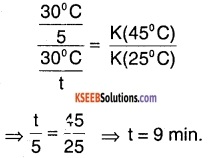∴ Time taken = 9 minutes.

### 1st PUC Physics Thermal Properties of Matter One Mark Questions and Answers

Question 1.
Define coefficient of thermal conductivity.
Coefficient of thermal conductivity of a material is defined as the quantity of heat conducted per second through a unit area of a slab of unit thickness when the temperature difference between its ends is 1K.

Question 2.
Heat radiation from a body due to its temperature is called thermal radiation.

Question 3.
What is a perfect black body?
A body, which absorbs all the radiation incident on it is called a perfect black body.

Question 4.
Define emissivity of a surface.
Emissivity of a surface is defined as the ratio of the quantity of thermal radiation emitted per sec per unit area of the surface to the quantity of thermal radiation emitted per sec per unit area of a perfect black body under identical conditions.Question 5.
Define absorptivity of a surface.
Absorptivity of a surface is defined as the ratio of the quantity of thermal radiation absorbed per sec to the quantity of radiation incident on it per second.

Question 6.
What is the unit of coefficient of thermal conductivity?
Wm-1K-1

Question 7.
What is the dimensional formula of coefficient of thermal conductivity?
MLT-3 θ-1

Question 8.
What happens to the heat supplied to a good conductor when it is under steady-state?
Heat is entirely conducted from the hot end to the cold end.

Question 9.
Why is coal tar heated before use?
Coal tar is heated to reduce its viscosity.Question 10.
What is the value of emissivity of a perfect black body?
Emissivity of a perfect black body is unity.

Question 11.
Give dimensions of solar constant and water equivalent.
Solar constant: [MT-3]
Water equivalent: [M]

### 1st PUC Physics Thermal Properties of Matter Two Marks Questions and Answers

Question 1.
Explain three methods of transfer of heat.

1. Conduction:
It is a process of transmission of heat in solids, without the actual movement of the particles.

2. Convection:
It is a process of transmission of heat in fluids (liquids and gases) with the actual movement of particles.

It is a process of transmission of heat without any medium.

Question 2.
When a solid is heated at one end, heat starts flowing from hot end to cold end. Heat supplied to any element of the solid is partly used in heating it, partly conducted through it and rest is radiated from its surface. After a certain stage, there is no variation of temperature with time and heat supplied is entirely conducted and radiated.

Such a state is called steady state. Under steady-state, the temperature at different parts of the solid remains constant and is independent of time. The ratio of the difference in temperature to the distance between two points in a heated body under steady state is called the temperature gradient.Question 3.
Mention the properties of thermal radiation.

1. It travels through a vacuum with the velocity of light.
2. It travels in a straight line.
3. It undergoes reflection, refraction and total internal reflection.
4. It exhibits the phenomenon of interference, diffraction and polarisation.
5. Intensity of radiation decreases with increase in distance.

Question 4.
Explain Ferry’s black body.Ferry’s black body consists of a hollow double-walled sphere with a fine hole and a pointed projection in front of the hole. The space between the two walls is evacuated to reduce any loss of radiation due to convection. The inner walls are coated with lamp black. Any radiation falling on the hole is completely absorbed due to multiple reflections, hence the hole acts as a black body.

Question 5.
State and explain Kirchhoff’s law.
At a given temperature, the ratio of the emissive power to the absorptive power for all bodies is a constant and is equal to the emissive power of a perfect black body.
If e is the emissive power and a is the absorptive power then, $$\frac{\mathrm{e}}{\mathrm{a}}$$ = constant  =1.
∴ e = a(∵ emissive power of a perfectly black body = 1)
Thus, the emissive power of a body is equal to its absorptive power, which means good emitter of thermal radiation is also a good absorber of heat.Question 6.
State and explain Newton’s law of cooling.
The rate of cooling of a body is directly proportional to difference in temperature of the body and the surrounding. If the temperature of a body
decreases from θ1 to θ2 in t seconds, then rate of cooling is, $$\frac{\theta_{1}-\theta_{2}}{t}$$
Average temperature of the body θ = $$\frac{\theta_{1}+\theta_{2}}{2}$$
According to Newton’s law,
$$\frac{\theta_{1}-\theta_{2}}{t} \propto\left(\theta-\theta_{0}\right)$$
θ0 – temperature of the surrounding.

Question 7.
State and explain Stefan’s law.
The total radiation emitted by a perfect black body per second per unit area of the surface is directly proportional to the fourth power of its absolute temperature. If E is radiation emitted per second per unit area of a perfect black body at temperature T then, E ∝ T4 or E = σT4 where s is called Stefan’s constant.

Question 8.
State and explain Wein’s displacement law.
The wavelength (λm) corresponding to maximum energy emitted by a black body is inversely proportional to its absolute temperature.
i. e., λm ∝ $$\frac{1}{T}$$ or λm T = constant.
The constant is called Wein’s Constant.

Question 9.
State and explain Planck’s law.
According to this law, radiation of a particular frequency (v) is emitted or absorbed in discrete amounts (E) given by the relation E = h v where h is a Planck’s constant. The value of h is 6.625 × 10-34 Js. These packets of energy are called quantum or photon.Question 10.
Define solar constant. What is its value?
The solar constant is defined as the amount of heat radiation absorbed normally per second per unit area of a perfect black body placed on the earth at a mean distance from the sun in the absence of atmosphere.
The value of solar Constant is 1350 W/m2.

Question 11.
State

2. State Stefan’s law of radiation

1. Kirchhoff’s law:
At a given temperature, the ratio of the emissive power to the absorptive power is a constant for all bodies and is equal to the emissive power of a perfect black body.

2. Stefan’s law:
The total radiation emitted by a perfectly black body per second per unit area is directly proportional to the 4th power of its absolute temperature.

Question 12.
Calculate the heat of combustion of coal, when 10 gm of coal, on burning raises the temperature of 2 litres of water from 20° C to 55° C.
mass of 2l of water = 2kg
∆T = 55° C – 20° C = 35° C
s = 4.2 × 103 J kg-1 °C-1
Heat produced by burning coal = ms ∆T
= 2 × 4.2 × 103 × 35
= 2.94 × 105 JQuestion 13.
2 kg of water at 80° C is mixed with 3 kg of water at 20°C. Assuming no heat losses, find the final temperature of the mixture.
m1 = 2 kg,
T1 = 80°C,
s = 4.2 × 103 J kg-1 °C-1,
m2 = 3kg,
T2 = 20° C
Let the final temperature be T3
Heat lost by 3 kg water = Heat gained by 2 kg water.
⇒ m1s(T1 – T3) = m2s(T3 – T2)
⇒ 2 × (80 – T3) = 3 × (T3 – 20)
⇒ T3 = 44° C
∴ Final temperature = 44° C

Question 14.
What are the basic requirements of a cooking utensil in respect of:

1. Specific heat and
2. thermal conductivity

For the temperature of the utensil to rise quickly, it is necessary for the utensil to have low specific heat. In order for the heat to spread quickly to the vegetables, the utensil must have high thermal conductivity.

### 1st PUC Physics Thermal Properties of Matter Three Marks Questions and Answers

Question 1.
State Wien’s displacement law. Draw the graph showing energy emitted versus wavelength for a black body at different temperatures.
Wein’s displacement law:
The wavelength (λm) Corresponding to maximum energy emitted by a black body is inversely proportional to its absolute temperature. i.e. λm ∝ $$\frac{1}{T}$$ or λm T = b. where b is a constant, called Wein’s constant. The radiation emitted by a black body is called black body radiation. The distribution of energy of a black body radiation at different temperatures is as shown in the figure.The energy distribution is not uniform. There is a particular wavelength λm at which the energy emitted is maximum. The wavelength λm for which the intensity is maximum decreases with increase in temperature.

Question 2.
Draw energy distribution curves for a black body at two different temperatures T1 and T2 (T1 > T2). Write any two conclusions that can be drawn from these curves.
The radiation emitted by a black body is called black body radiation. The distribution of energy of a black body radiation at different temperatures with its wavelength is as shown in the figure.Question 3.
Draw labelled curves which show how the energy in the black body radiation spectrum varies with wavelength at various temperatures. What result does this lead to?
The radiation emitted by a black body is called black body radiation. The distribution of energy of a black body radiation at different temperatures with its wavelength is as shown in the figure.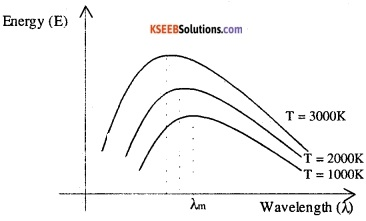### 1st PUC Physics Thermal Properties of Matter Four Or Five Marks Questions and Answers

Question 1.
State and explain the laws of thermal conductivity.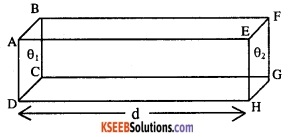Consider a metal slab of area of cross-section A and thickness d. Let the end faces (ABCD & EFGH) be maintained at temperatures θ1 and θ21 > θ2).
The amount of heat conducted (Q) from the hotter to colder face is,
1. directly proportional to the area of cross-section (A)
2. directly proportional to the time for which heat flows (t)
3. directly proportional to the temperature difference between the faces (θ1 – θ2)
4. inversely proportional to the distance between the faces (d)
This is called the law of thermal conductivity.
According to the law Q ∝ $$\frac{\mathrm{At}\left(\theta_{1}-\theta_{2}\right)}{\mathrm{d}}$$
$$\mathrm{Q}=\frac{\mathrm{KAt}\left(\theta_{1}-\theta_{2}\right)}{\mathrm{d}}$$
K is a constant of proportionality called coefficient of thermal conductivity.

Question 2.
What is black body radiation? Explain the characteristics of It.
The radiation emitted by a black body is called black body radiation. The distribution of energy of a black body radiation at different temperatures with its wavelength is as shown in the figure.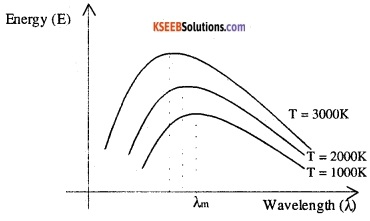1. A black body emits radiation of all wavelengths lying in the region of ultraviolet, visible light and infrared.
2. As the temperature of the black body increases, the total amount of radiation emitted by it increases. (According to Stefan’s law)
3. The energy distribution is not uniform. There is a particular wavelength λm at which the energy emitted is maximum.
4. The wavelength λm for which the energy emitted is maximum decreases with increase in temperature.Question 3.It consists of a concave mirror M made of copper and plated with nickel. The mirror has a small hole at the centre at which an eyepiece E is fitted. A diaphragm D is placed at the focus of M; which has a hole to allow radiation to pass through it. A blackened metal strip S is placed behind D. To the other surface of S, one junction of a thermocouple TT is connected. The thermocouple is connected to a millivoltmeter. A screen R is used to protect the strip from direct radiation. The mirror can be adjusted for focusing.

Question 4.
Why is ice packed in sawdust? Explain.
Ice is packed in sawdust because it prevents ice from melting. Sawdust is a bad conductor of heat.

Question 5.
Explain the different modes of transmission of heat. Explain Wein’s displacement law with the help of graph of intensity versus wavelength.
1. Conduction:
It is a process of transmission of heat in solids, without the actual movement of the particles.
Eg: When metal rod is heated at one end, after some time another end also becomes hot.

2. Convection:
It is a process of transmission of heat in fluids (liquids and gases) with the actual movement of particles.
Eg: Smoke carries heat energy from the flame by convection.

It is a process of transmission of heat without any medium in the form an electromagnetic waves.
Eg: Heat from the sun reaches the earth by radiation.

Wein’s displacement law:
The wavelength (λm) Corresponding to maximum energy emitted by a black body is inversely proportional to its absolute temperature. i.e. λm ∝ $$\frac{1}{T}$$ or λm T = b. where b is a constant, called Wein’s constant. The radiation emitted by a black body is called black body radiation. The distribution of energy of a black body radiation at different temperatures is as shown in the figure.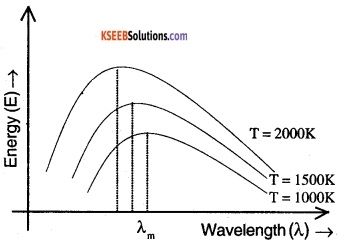The energy distribution is not uniform. There is a particular wavelength λm at which the energy emitted is maximum. The wavelength λm for which the intensity is maximum decreases with increase in temperature.

Question 6.
What is a black body? Draw the curves showing the energy distribution among black body radiations at different temperature. Hence define Wein’s displacement law. Give one application of Wein’s displacement law.
1. black body:
The radiation emitted by a black body is called black body radiation. The distribution of energy of a black body radiation at different temperatures with its wavelength.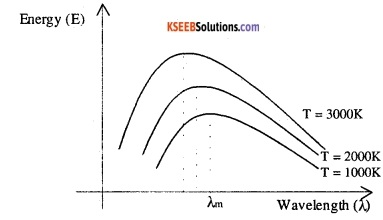Wein’s displacement law:
The wavelength (λm) Corresponding to maximum energy emitted by a black body is inversely proportional to its absolute temperature. i.e. λm ∝ $$\frac{1}{T}$$ 17 or λm T = b. where b is a constant, called Wein’s constant.Question 7.
Discuss briefly energy distribution of black body radiation. Hence deduce Wien’s displacement law and Stefan’s law.
Wein’s displacement law:
The wavelength (λm) Corresponding to maximum energy emitted by a black body is inversely proportional to its absolute temperature. i.e. λm ∝ $$\frac{1}{T}$$ or λm T = b. where b is a constant, called Wein’s constant. The radiation emitted by a black body is called black body radiation. The distribution of energy of a black body radiation at different temperatures is as shown in the figure.The energy distribution is not uniform. There is a particular wavelength λm at which the energy emitted is maximum. The wavelength λm for which the intensity is maximum decreases with increase in temperature.

Stefan’s law:
The total radiation emitted by a perfect black body per second per unit area of the surface is directly proportional to the fourth power of its absolute temperature. If E is radiation emitted per second per unit area of a perfect black body at temperature T then, E ∝ T4 or E = σT4 where s is called Stefan’s constant.

1st PUC Physics Thermal Properties of Matter Numerical Problems Questions and Answers

Question 1.
A circular plate has a radius of 10 cm and thickness 1 cm. If one face of the plate is kept in contact with steam and the other face is in contact with ice, calculate the amount of heat flowing per hour across the plate. K as 85 W-1m-1K-1.
Solution:
The amount of heat conducted in t seconds is given by,
$$Q=K \frac{A\left(\theta_{1}-\theta_{2}\right) t}{d}$$
Here, K = 85 W m-1K-1
A = π r2 = 3.14 × (0.1)2
t = 1 hr = 60 × 60 s.
d = 10-2 m2
1 – θ2) = 373 -273 = 100 K
Q= $$\mathrm{K} \frac{\mathrm{A}\left(\theta_{1}-\theta_{2}\right) \mathrm{t}}{\mathrm{d}}$$
= $$\frac{85 \times 3.14 \times(0.1)^{2} \times 100 \times 60 \times 60}{10^{-2}}$$
= 96.08×106 J.

Question 2.
A metal rod 0.314 m long and 0.02 m in diameter has one end in boiling water at 373K and the other end in melting ice at 273K. Find the amount of ice that will melt per hour? K = 385 Wm-1K-1.
Latent heat of Ice = 3.36 × 105 Jkg-1.
Solution:
If ‘m’ is mass of ice that melts in one hour, then quantity of heat conducted is Q = mL
But Q = K $$\frac{A\left(\theta_{1}-\theta_{2}\right) t}{d}$$
∴ Q = mL
m = $$\frac{\mathrm{KA}\left(\theta_{1}-\theta_{2}\right) t}{\mathrm{d} \times L}$$
Here, K =385Wm-1K-1
A = p r2 =3.14 × (0.01 )2
L = 3.3 × 105 Jkg-1
θ1 – θ2 = 373 – 273 = 100 K
t = 1 hour = 3600 s
d = 0.314 m
∴ m= $$\frac{385 \times 3.142 \times(0.01)^{2} \times 100 \times 60 \times 60}{0.314 \times 3.36 \times 10^{5}}$$
= 41.28 x 10-2kg

Question 3.
Two metal rods of the same area of cross-section and with their lengths in the ratio 1:3 are joined together to form a compound rod. One end of this compound rod is kept at 100°C and the other at 0° C. Calculate the temperature at the Junction if the thermal conductivity is in the ratio 1:3.
Solution:Let AB and BC be the two rods of length L1 and L2 and having the same area of cross-section A, joined together to form a compound rod as shown in the figure.
If ‘ θ ’ is the steady temperature at the junction B during the steady-state, then, rate of flow of heat through AB = rate of flow through BC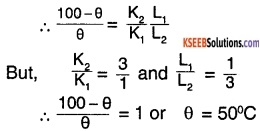Question 4.
Calculate rate of heat radiated per unit area from the surface of a furnace at a temperature 2000K, assuming it to be a black body. If the emissivity is 0.37, then what Is the amount of heat radiated per second? G =5.67×10-8Wm-2K-4.
Solution:
From Stefan’s law of radiation, the amount of heat radiated per second is E = σT4.
E =5.67 × 10-8 × (2000)4
= 5.67 × 24 × 104
= 5.67 × 16 × 104
= 9.072 × 105Js-1.
If e is the emissivity of the surface, then the heat radiated per second R1 = eR
= 0.37 × 9.072 × 105
= 3.35 × 105 Js-1Question 5.
A slab of stone of area 0.36m2 and thickness 0.1m is exposed on the lower surface to steam at 373 K. A block of ice at 273 k rests on the upper surface of the slab. If in one hour, 4.8kg of Ice is melted, calculate the conductivity of the stone. Latent heat of Ice = 3.35 x 105 JK-1.
Solution:
A = 0.36m2,
d = 0.1m
θ1 = 373 K
θ2 = 273 K
t = 1 hr = 3600s,
m = 4.8kg,
K = ?
L = 3.35 × 105 Jkg-1
Heat conducted through the rod = Heal used in melting ice
$$\frac{\mathrm{KA}\left(\theta_{1}-\theta_{2}\right) \mathrm{t}}{\mathrm{d}}$$ = mL
K = $$\frac{m L d}{A\left(\theta_{1}-\theta_{2}\right) t}=\frac{4.8 \times 3.35 \times 10^{5} \times 0.1}{0.36 \times 100 \times 3600}$$
= 1.241 Wm-1 K-1.

Question 6.
The filament of 40 W electric lamp of length 0.1m and diameter 0.1mm is kept at a temperature of 2773 K. Calculate the emissive power if all the heat is lost by radiation. σ = 5.67 × 10-8 Wm-2 K-4].
Solution :
P = 40 W,
l = 0.1 m,
r = 0.05 mm
T = 2773 K
e = ?
Area = 2π rl =3.142 x 10-5 m2
Energy emitted by a source in t sec
E = e A σ T4t
$$\frac{E}{t}$$ = e A σ T4
P = e A σ T4
e = $$\frac{P}{A \sigma T^{4}}$$
$$=\frac{40}{3.142 \times 10^{-5} \times 5.67 \times 10^{-8} \times(2773)^{4}}$$
= 0.3798

Question 7.
The thickness of ice in a lake at a certain moment is 0.03m. At what rate the thickness of the ice increasing and how long will it take for the thickness of ice layer to be doubled, if the temperature of air is -20° C. Given coefficient of thermal conductivity of ice=0.168 Wm-1K-1 degree. Latent heat of fusion of ice=3.35 × 10 Jkg-1 density of ice= 9.2 × 102 Kg/m3
Solution:
When the layer of ice covers the lake, the lower part of the ice is in contact with ice is at 0°c and outer surface with air at – 20°c
∴ θ1 =0°c and θ2 = – 20°c
Let the thickness of ice increase by small amount ∆x by an area A in a time ∆t.
Then,
mass of ice formed = A × ∆x × ρ
Quantity of heat, Q = A × ∆x × ρ × L    …..(1)
But, Q = $$\frac{\mathrm{k} \mathrm{A}\left(\theta_{1}-\theta_{2}\right) \Delta \mathrm{t}}{\mathrm{d}}$$ ….(2)
from equation (1) & equation (2)
A × ∆x × r × L = $$\frac{\mathrm{k} \mathrm{A}\left(\theta_{1}-\theta_{2}\right) \Delta \mathrm{t}}{\mathrm{d}}$$
$$\frac{\Delta x}{\Delta t}=\frac{k A\left(\theta_{1}-\theta_{2}\right)}{d x A x \rho x L}$$
$$=\frac{0.168 \times 20}{0.03 \times 9.2 \times 10^{2} \times 3.35 \times 10^{5}}$$
= 3.634 × 10-7ms-1
The time taken for the ice layer to double is
t = $$\frac{\Delta x / \Delta t}{d}$$
$$=\frac{3.634 \times 10^{-7}}{0.06}$$
= 60.57 × 10-7secQuestion 8.
Estimate the rate at which ice melts in a wooden box 0.04m thick, of inside measurement 0.6 m × 0.4m × 0.4m. Assume that the outside temperature is 300 K and the conductivity of wood is 0.08 wm-1K-1
Solution:
Area of the box
= A = 4 × (0.6 × 0.4) + 2 × (0.4 × 0.4)= 1.28m2
d = 0.04m
k= 0.08wm-1K-1
L= 3.36 × 105Jkg-1
Let Q be the heat transmitted per second.
Then, Q = $$\frac{\mathrm{k} \mathrm{A}\left(\theta_{1}-\theta_{2}\right) \mathrm{t}}{\mathrm{d}}$$
$$=\frac{0.08 \times 1.28 \times(300-273) \times 1}{0.04}=69.12 J$$
To melt 1 kg of ice, 3.36 × 105J of heat is required
∴ Rate at which ice melts
$$=\frac{69.12}{3.36 \times 10^{-5}}$$
= 20.57 × 10-5kgs-1.

Question 9.
A man wraps himself in flannel 4 mm thick. How much heat will be lost per square metre of his body per hour if the atmosphere temperature is 20° and the body temperature is 37°c(The co-efficient of thermal conductivity of flannel is 0.05 Wm-1k-1)
Solution:
k=0.05Wm-1k-1
d = 4mm = 4 × 103
m = 0.004m
θ1 =37°c,
θ2 =20°c
t =1 hr=3600 seconds
A =1m2
The quantity of heat conducted is
$$Q=\frac{k A\left(\theta_{1}-\theta_{2}\right) t}{d}$$
$$=\frac{0.05 \times 1(37-20) 3600}{0.004}$$
= 7.65 × 105J

Question 10.
Calculate the rate at which heat is lost through a glass window of area 0.1m2 thickness 4mm when the temperature inside is at 40° c and outside is -5°c. Co-efficient of thermal conductivity of a glass is 1.1wm-1k-1.
Solution:
A=0.1m2
d = 4mm = 4 × 10-3
m=0.004m
θ1 = 40°C
θ2 = -5°c
k = 1.1Wm-1k-1
Heat lost through the glass window is
$$Q=\frac{K A\left(\theta_{1}-\theta_{2}\right) t}{d}$$
$$=\frac{1.1 \times 0.1(40+5) \times 1}{0.004}$$
= 1237.5J

Question 11.
The two faces of metal plate of thickness 40mm and area 102m2 maintained at 273K and 373K. The coefficient of thermal conductivity of the metal is 380 Wm-1k-1 Find the quantity of heat flows, through the plate in one hour.
Solution:
d = 40mm = 40 × 10-3
m = 0.04m
A = 102m2
θ2 = 273k
θ1 =37K
K = 380Wm-1k-1
t = 1hr = 3600 seconds
The quantity of heat that flows in one hour is
$$Q=\frac{k A\left(\theta_{1}-\theta_{2}\right) t}{d}$$
$$=\frac{380 \times 10^{2}(373-273) \times 3600}{0.04}$$
Q = 3.42 × 1011J

Question 12.
A spherical body of radius 0.01m is at 400K. Calculate the energy radiated by the body per second per unit area. Assume the body to be perfect black body.
Solution:
From stefan’s law of radiation, amount of heat radiated per second per unit area is
E = σ T4
= 5.67 × 10-8 × (400)4 = 1451.5s-1
The total energy radiated per second is
E = A σ T4
E = P r2 σ T4
= 3.142 × (0.01 )2 × 5.67 × 10-8 × (400)4
= 0.4561s-1

Question 13.
A metal rod 0.3m long & 0.02m in diameter has one end at 100°C & other end at 0°C. Calculate the total amount of heat conducted in 2 minutes. Given k = 385 JS-1m-1K-1.
Solution:
l = 0.3m,
d = 0.02m
∴ r = 0.01m
θ1 = 100°C
θ2 = O°C
t = 2 minutes = 120 S
k = 385 JS-1m-1K-1.
Q = ?
t= π r2 = 3.142 × 0.012 = 3.142 × 104m2
$$\therefore Q=\frac{k A t\left(\theta_{1}-\theta_{2}\right)}{\ell}$$
$$=\frac{385 \times 3.142 \times 10^{4} \times 120(109)}{0.3}$$
= 4.8 × 103 JQuestion 14.
A metallic rod 1.5m long and 2×104 m2 in area is heated at one end. The coefficient of thermal conductivity of the material of the metallic rod is 210 Js-1m-1K-1. In the steady-state the temperature of the ends of the rod are 373K and 263 K. Calculate.

1. Temperature gradient In the rod.
2. Temperature at a point 0.8 m from the hot end.
3. Rate of heat transmission.

d=1.5m,
A = 2 × 104m2,
K = 210 W/m/k, θ1 = 373K and θ2 = 263K.
$$\frac{\theta_{1}-\theta_{2}}{d}=\frac{373-263}{1.5}=\frac{110}{1.5}=73.33 \mathrm{K} / \mathrm{m}$$

2. Let θ be the temperature at a distance d1 = 0.8m from the hot end then temperature gradient = $$\frac{\theta_{1}-\theta}{d^{1}}$$
i.e., 73.33 = $$\frac{373-\theta}{0.8}$$ or θ =314.336 K.

3. Rate of heat transmission
$$\frac{\mathrm{q}}{\mathrm{t}}=\mathrm{KA}\left(\frac{\theta_{1}-\theta_{2}}{\mathrm{d}}\right)$$
= 210 × 2 × 10-4 × 73.33
= 3.08 J/s.

Question 15.
The two faces of a metal plate of thickness 40 mm and area 10-2m2 are maintained at 273 K and 373 K. The coefficient of thermal conductivity of metal is 380 w/m/K. Find the quantity of heat that flows through the plate in one hour.
d = 40 × 10-3m,
A = 10-2m2,
t = 1 hr = 3600s,
θ1 = 373K,
θ1 = 2.73K and K= 380 W/m/K
$$\mathrm{q}=\frac{\kappa \mathrm{At}\left(\mathrm{q}-\theta_{2}\right)}{\mathrm{d}}$$
= 34.6×106J.

Question 16.
The time taken for a substance to cool form 100° C to 50° C is 5 minutes. If the room temperature is 30°C, what time required for same substance to cool from 50°C to 40°C?
Case (i):
Rate of cooling
$$\frac{\mathrm{d} \theta}{\mathrm{dt}}=\frac{100-50}{5}$$ = 10° C/min,
Average temperature
$$\theta=\frac{100+50}{2}=75^{\circ} \mathrm{C}$$
Room temperate θ0 = 30°C.
According to Newton’s law of cooling,
$$\frac{\mathrm{d} \theta}{\mathrm{dt}}$$ = -K(θ – θ0).
10 = -K(75 – 30).
K= $$-\frac{10}{45} / \mathrm{min}$$ . ……(1).

Case(ii):
Rate of cooling
$$\frac{\mathrm{d} \theta}{\mathrm{dt}}$$ = $$\frac{50-40}{\mathrm{dt}}=\frac{10}{\mathrm{dt}}$$,
Average temperature
$$\theta=\frac{50+40}{2}=45^{\circ} \mathrm{C}$$
Room temperate e θ0 = 30°C.
According to Newton’s law of cooling,
$$\frac{\mathrm{d} \theta}{\mathrm{dt}}$$ = K(θ – θ0)
$$\frac{10}{\mathrm{dt}}=-\left(\frac{-10}{45}\right)(45-30)$$ (from)
dt =3 min.

1st PUC Physics Thermal Properties of Matter Hard Questions and Answers

Question 1.
A hot body placed in air is cooled down. According to Newton’s law of cooling, the rate of decrease of temperature being ‘k’ times the temperature of the surrounding. Starting from t = 0, find the time in which the body will lose 90% of the maximum heat it can lose.
We have,
$$\frac{\mathrm{d} \mathrm{T}}{\mathrm{dt}}$$ = – K(T – T0)
where T0 is the temperature of the surrounding and T is the temperature of the body at time t. Let T = T1 at t = 0.
Then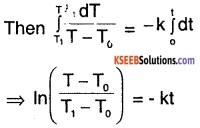⇒ T – T0 = (T1 – T0) e-kt ……(1)
The body continues to lose heat until its temperature becomes equal to that of the surrounding. The loss of heat in this entire period is
∆H = ms(T -T0).
This is the maximum heat it can lose. If the body loses 90% of this heat, the decrease in to temperature will be
$$\frac{9 \Delta \mathrm{H}}{10 \mathrm{ms}}$$ = $$\frac{9}{10}$$ (T1 – T0)
If the body loses this heat in time t, the temperature at t1 will be
T1 – $$\frac{9}{10}$$ (T1 – T0)
$$=\frac{T_{1}}{10}+\frac{9 T_{0}}{10}$$
Putting these values of time and temperature in (1),
$$\frac{T_{1}+9 T_{0}}{10}$$ – T0 = (T1 – T2) e-kt1
⇒ $$\frac{T_{1}-T_{0}}{10}$$ = (T1 – T2) e-kt1
⇒ e-kt1 = 1/10
⇒ t1 = $$\frac{\ln 10}{k}$$
∴ Required time = $$\frac{\ln 10}{k}$$Question 2.
Two thin metallic spherical shells of radii r1 and r2 (r1 < r2 are placed with their centres coinciding. A material of thermal conductivity k is filled in the space between the shells. The inner shell is maintained at temperature T1 and the outer shell at temperature T2 (T1 < T2). Calculate the rate at which heat flows radially through the material.